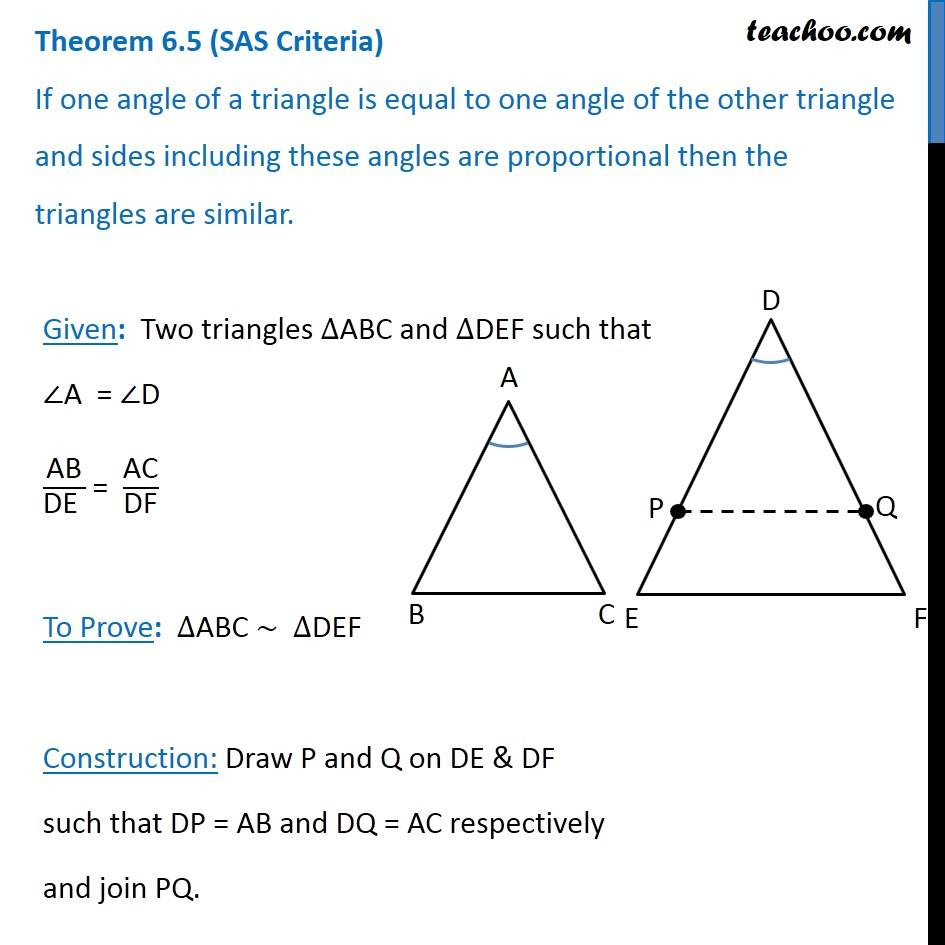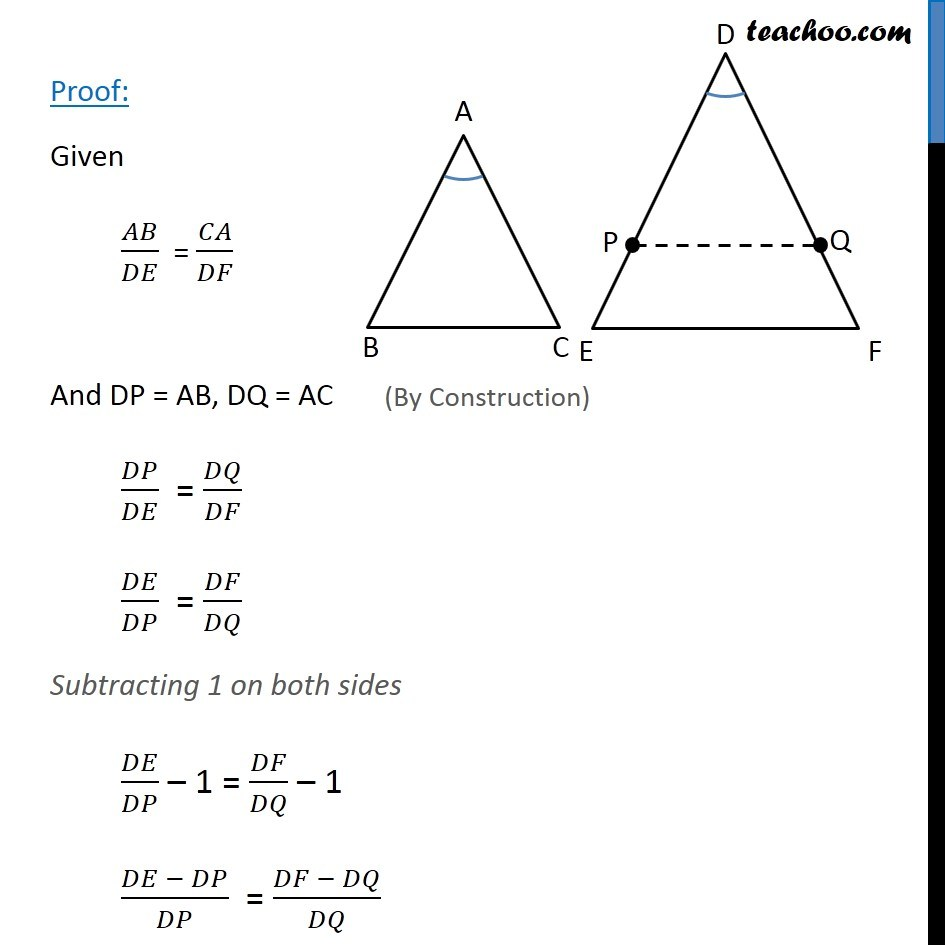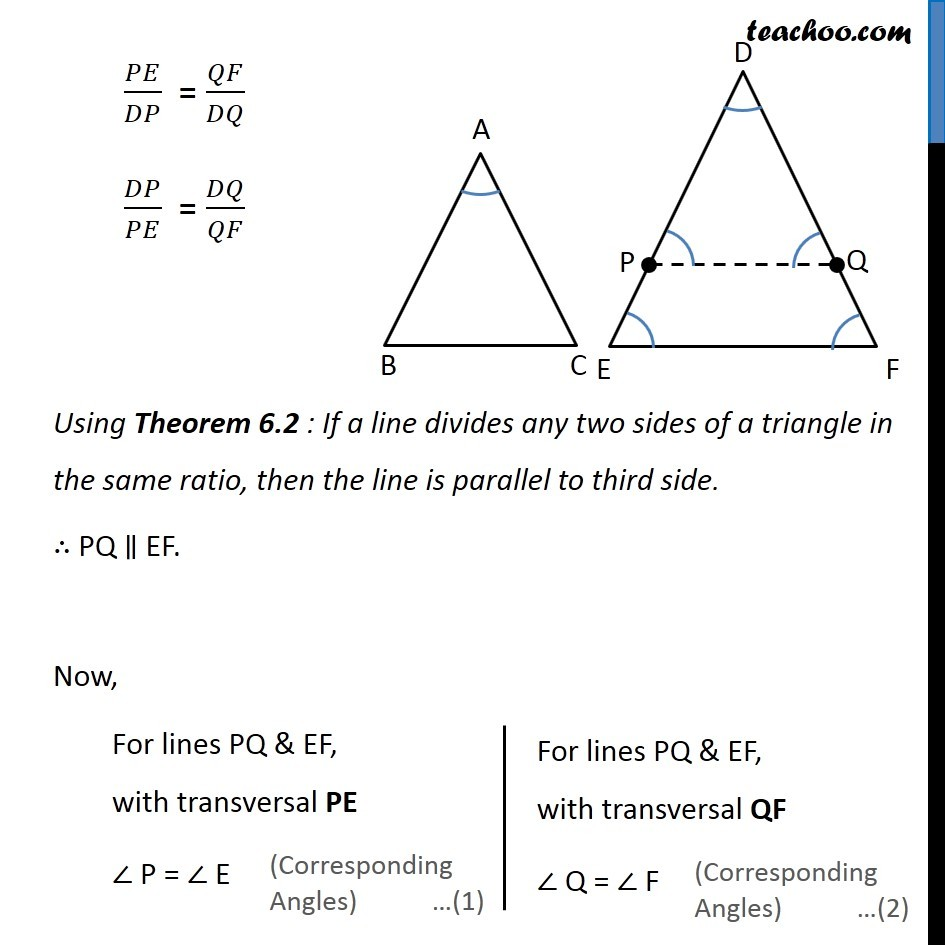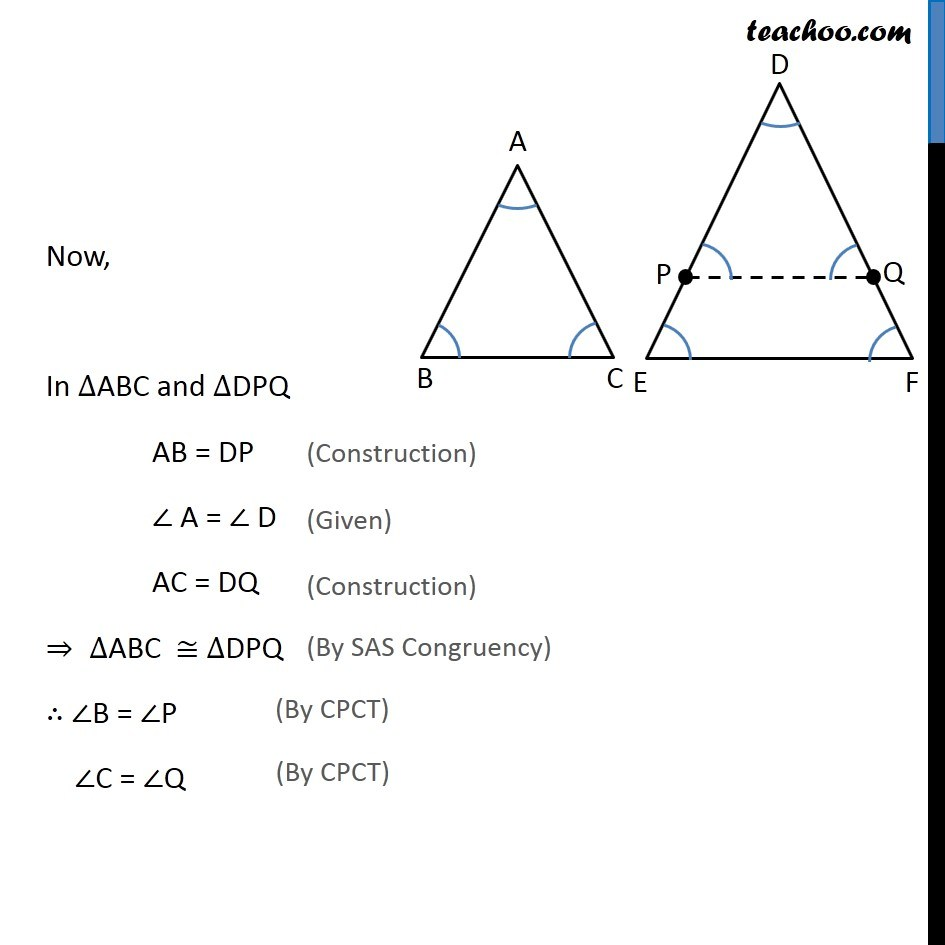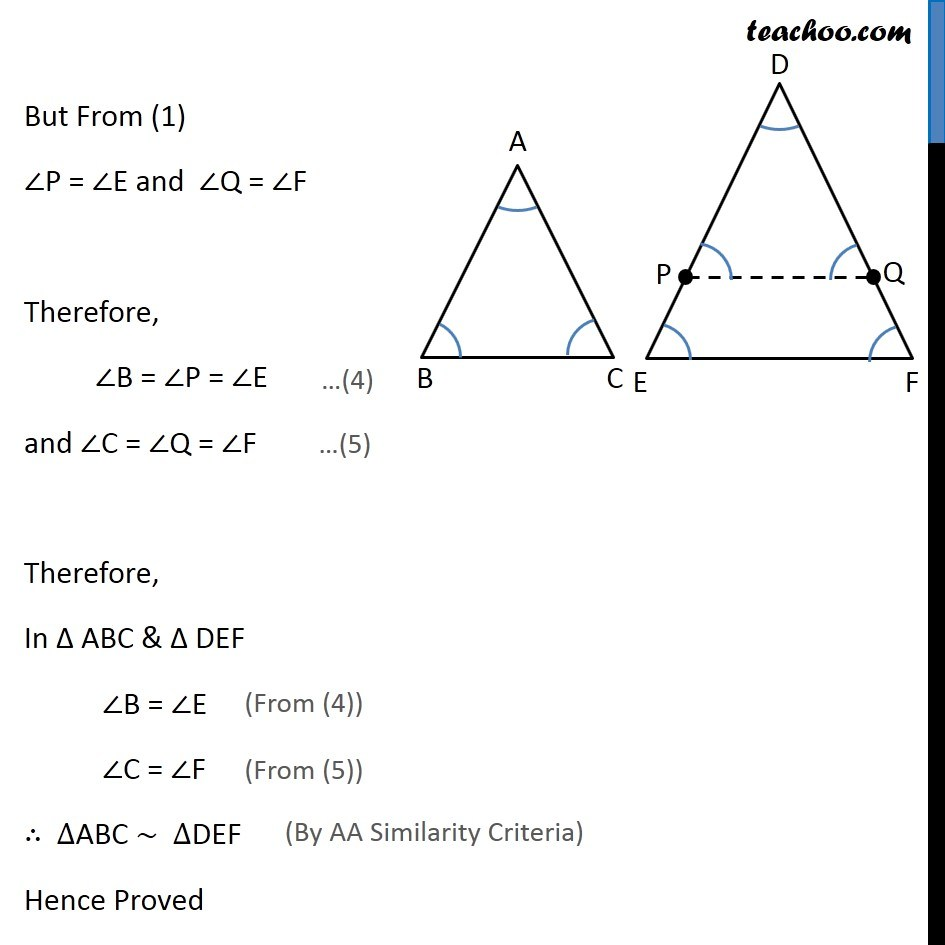Subscribe to our Youtube Channel - https://you.tube/teachoo

1. Chapter 6 Class 10 Triangles
2. Concept wise
3. SAS Similarity

Transcript

Theorem 6.5 (SAS Criteria) If one angle of a triangle is equal to one angle of the other triangle and sides including these angles are proportional then the triangles are similar. Given: Two triangles ∆ABC and ∆DEF such that ∠A = ∠D "AB" /"DE " = "AC" /"DF" To Prove: ∆ABC ~ ∆DEF Construction: Draw P and Q on DE & DF such that DP = AB and DQ = AC respectively and join PQ. Proof: Given 𝐴𝐵/𝐷𝐸 = 𝐶𝐴/𝐷𝐹 And DP = AB, DQ = AC 𝐷𝑃/𝐷𝐸 = 𝐷𝑄/𝐷𝐹 𝐷𝐸/𝐷𝑃 = 𝐷𝐹/𝐷𝑄 Subtracting 1 on both sides 𝐷𝐸/𝐷𝑃 – 1 = 𝐷𝐹/𝐷𝑄 – 1 (𝐷𝐸 − 𝐷𝑃)/𝐷𝑃 = (𝐷𝐹 − 𝐷𝑄)/𝐷𝑄 𝑃𝐸/𝐷𝑃 = 𝑄𝐹/𝐷𝑄 𝐷𝑃/𝑃𝐸 = 𝐷𝑄/𝑄𝐹 Using Theorem 6.2 : If a line divides any two sides of a triangle in the same ratio, then the line is parallel to third side. ∴ PQ ∥ EF. Now, For lines PQ & EF, with transversal PE ∠ P = ∠ E For lines PQ & EF, with transversal QF ∠ Q = ∠ F Now, In ∆ABC and ∆DPQ AB = DP ∠ A = ∠ D AC = DQ ⇒ ∆ABC ≅ ∆DPQ ∴ ∠B = ∠P ∠C = ∠Q But From (1) ∠P = ∠E and ∠Q = ∠F Therefore, ∠B = ∠P = ∠E and ∠C = ∠Q = ∠F Therefore, In Δ ABC & Δ DEF ∠B = ∠E ∠C = ∠F ∴ ∆ABC ~ ∆DEF Hence Proved

SAS Similarity